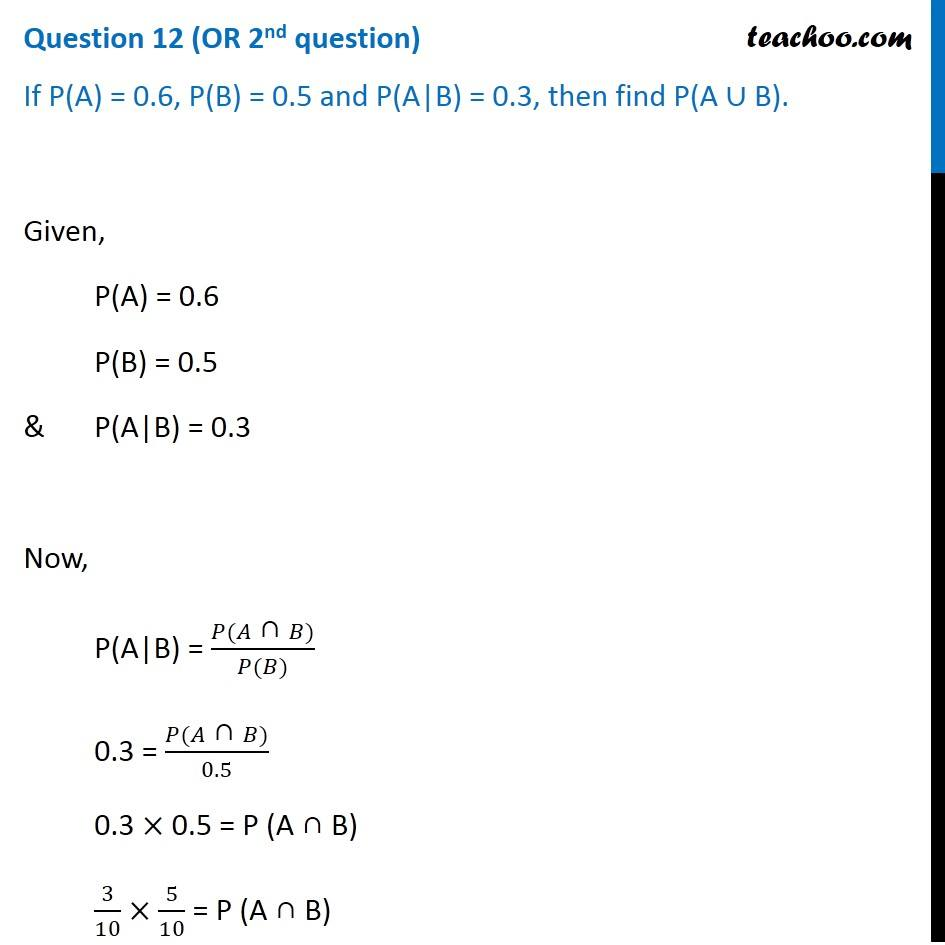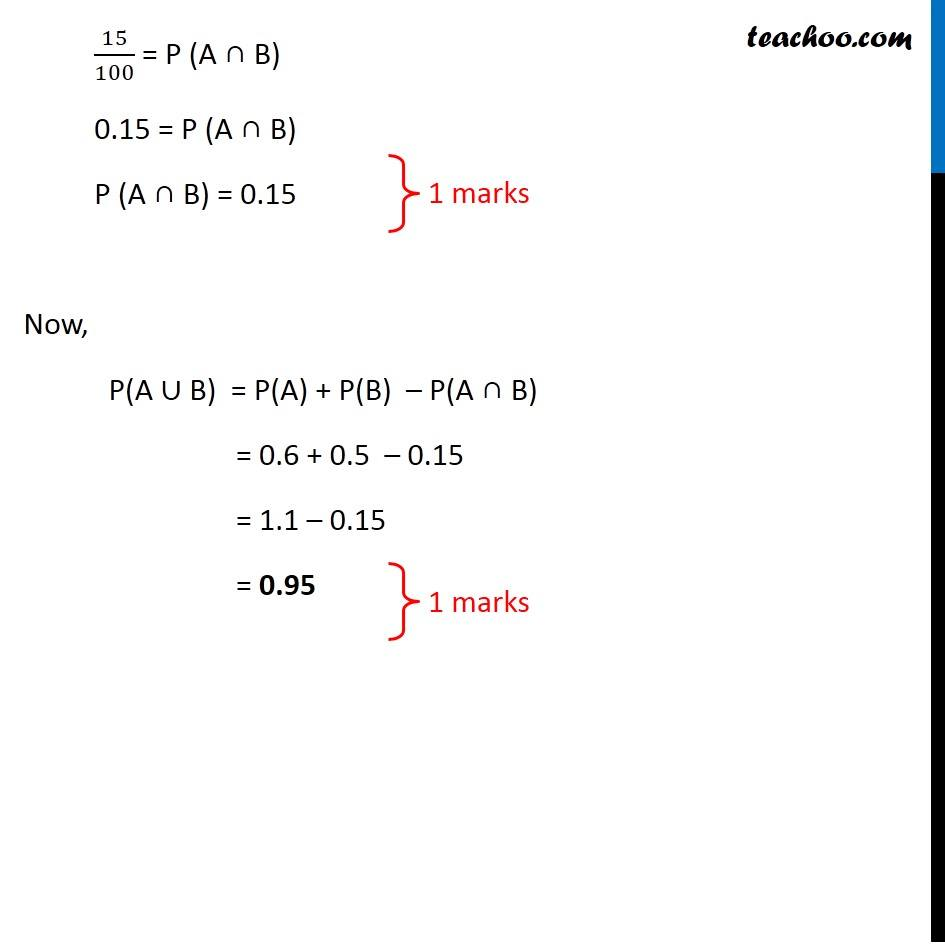CBSE Class 12 Sample Paper for 2019 Boards

Class 12
Solutions of Sample Papers and Past Year Papers - for Class 12 Boards

Question 12 (OR 2 nd question)

If P(A) = 0.6, P(B) = 0.5 and P(A|B) = 0.3, then find P(A ∪ B).Get live Maths 1-on-1 Classs - Class 6 to 12

### Transcript

Question 12 (OR 2nd question) If P(A) = 0.6, P(B) = 0.5 and P(A|B) = 0.3, then find P(A ∪ B). Given, P(A) = 0.6 P(B) = 0.5 & P(A|B) = 0.3 Now, P(A|B) = (𝑃(𝐴" ∩ " 𝐵))/(𝑃(𝐵)) 0.3 = (𝑃(𝐴" ∩ " 𝐵))/0.5 0.3 × 0.5 = P (A ∩ B) 3/10 × 5/10 = P (A ∩ B) 15/100 = P (A ∩ B) 0.15 = P (A ∩ B) P (A ∩ B) = 0.15 Now, P(A ∪ B) = P(A) + P(B) – P(A ∩ B) = 0.6 + 0.5 – 0.15 = 1.1 – 0.15 = 0.95